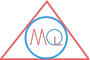# Point, Line, Ray, Line Segment and Plane

## What is a Point in Geometry?

In geometry, when we mark the exact position of an object with a dot, that is called a point. It has no length and breadth. Also, it occupies no depth. In other words, a point determines a location.

It is denoted by a dot “$$\cdot$$” symbol.

We use capital letters of alphabets to name a point.

Example

$$\cdot$$ A (read as point A)

$$\cdot$$ X (read as point X)

Two points A and X## What is a Line in Geometry?

A Line is one dimensional figure which is straight, has indefinite length and no thickness. Line can be extended indefinitely only in two directions which are opposite to each other. A line has infinite number of points lying on it.

A line has no end points.

We can name the lines by using two capital letters of alphabets and an arrow that points in both directions.

Example

$$\overleftrightarrow{AB}$$

Line AB## What is a Ray in Geometry?

It is a straight line which starts from one fixed point and always progress in one direction only away from that starting point. Ray can be extended indefinitely only in one direction.

It has one end point. It has no definite length and can’t be measured.

Ray is represented by a two capital letters of alphabets with a pointed arrow on top of it.

Example

$$\overrightarrow{AB}$$

where, A is the starting fixed point of ray.

Ray AB## What is a Line Segment in Geometry?

A Line segment is a part or segment of a line that is bounded by two distinct end points those lie on that line. A Line segment has number of other points lying on it, but they all lie in between the two end points only.

Line segment is also represented by two capital letters of alphabets but with a line on top of it.

Example

$$\overline{AB}$$

Line segment AB## What is a Plane in geometry?

In geometry, a plane is a flat and smooth surface. It has length and width. It has no thickness. Plane can be extended indefinitely in all directions.

Example of a PlaneWe can name the plane by using English alphabets. For example, the plane drawn in the above diagram can be named with letters A, B and C and called as Plane ABC.

Another example of a PlaneThis plane can be named with letters P, Q, R and S and called as Plane PQRS.

The real life examples of a plane, that can we see around us and everywhere are surface of a wall and ceiling of a room.

## What are Intersecting lines?

Lines are said to be intersecting lines if they meet out each other at a point.

Intersecting lines AB and CDLine AB and line CD intersect each other at a point E.

## What are Perpendicular lines?

The lines are said to be perpendicular lines if they intersect each other and the angle between them is $$90^0$$.

Perpendicular lines AB and CDLines AB and CD intersect at O and form an angle of $$90^0$$ in each quadrant.

Line AB is perpendicular to line CD. To denote AB is perpendicular to CD, we use symbol $$\bot$$.

So, we can write it as $$AB \bot CD$$ or we can write as $$CD \bot AB$$.

## What are Parallel lines?

Two straight lines are said to be parallel lines if they do not intersect each other at any point although we extend these line in both directions indefinitely.

Parallel lines AB and CDLine AB is parallel to CD. To denote it, we can use symbol $$||$$. So. it is written as $$AB||CD$$ or $$CD||AB$$.

## What is a Transversal line?

A line is said to be a transversal line, if it cuts two or more lines at different points and those lines can be parallel or non parallel lines.

Transversal lines AB and CDHere, line AB cuts through two lines PQ and RS where line AB is called as transversal line.

## What are Concurrent lines?

If three or more straight lines pass through the same point or intersect each other at same point, then these lines are called concurrent lines.

The point where lines intersect each other, is called point of concurrence.

Concurrent lines AB, CD, PQ and RSHere, AB, CD, PQ and RS are concurrent lines, as these lines pass through the same point T. T is called the point of concurrence.

### Q) What is a point?

A point is a mark of a position.

### Q) What is a line?

A line refers to a straight line which can be extended indefinitely in both directions.

### Q) What is a plane?

Plane is a flat surface which can be extended indefinitely in all directions.

## Solved Examples

### 1) Identify ray and line segment from the following figure.Solution

Ray is $$\overrightarrow{AC}$$

Line segment is $$\overline{AB}$$

### 2) Draw a line m and name a line segment AB on line m.

Solution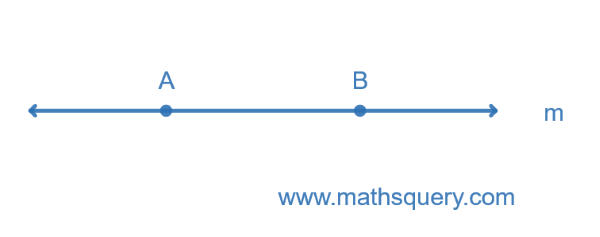Here, m is a line and AB is a line segment on it.

### 3) Check whether following pairs of lines are parallel, intersecting, concurrent or perpendicular?

1.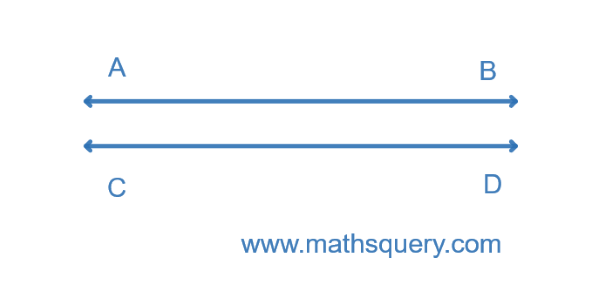2.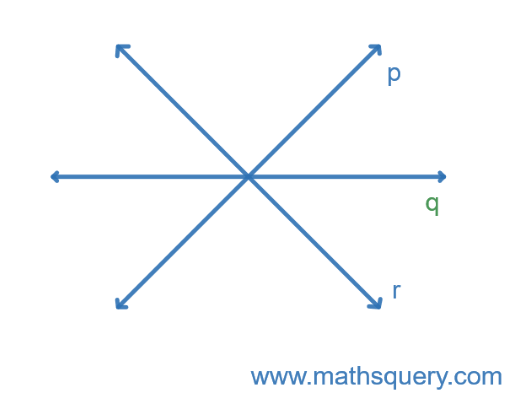3.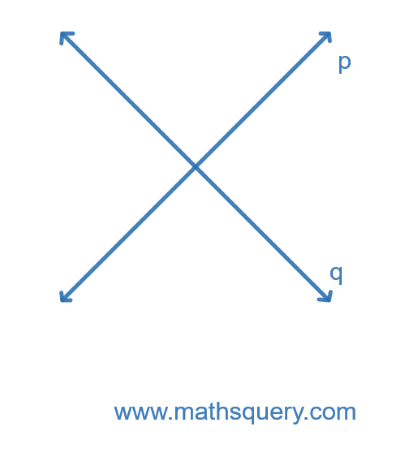4.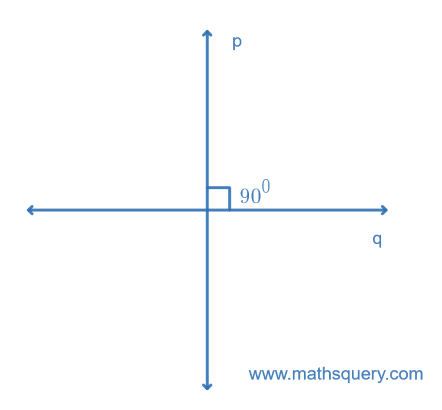Solution

a. Parallel lines

b. Concurrent lines

c. Intersecting lines

d. Perpendicular lines

### 4) How many line segments are there in the following figure?Solution

PQ, PR, PS, QR, QS and RS are six line segments.

## Multiple Choice Questions

### 1) A point has no

1. length
2. width
3. thickness
4. All of above

### 2) A line has only

1. length
2. width
3. thickness
4. All of above

### 3) Number of points lying on a line is

1. finite
2. countless
3. fixed
4. All of above

### 4) Example of parallel lines is

1. rail lines
2. opposite edge of table
3. opposite edge of ruler
4. All of above

### 5) A plane is a flat surface which has

1. length
2. width
3. no thickness
4. All of above

### 6) The intersection of two planes is a

1. straight line
2. curve
3. plane
4. None of these

### 7) If two lines have one common point, then these lines are called

1. concurrent lines
2. intersecting lines
3. parallel lines
4. Transversal lines

### 8) A line segment has two end points but a line has

1. no end point
2. one end point
3. two end points
4. three end points

### 9) If three or more points lie on the same straight line, then points are called as

1. point of concurrence
2. point of intersection
3. collinear points
4. mid point

1. 00
2. 1800
3. 900
4. 1200